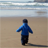Announcements
You can succeed best and quickest by helping others to succeed. Join the conversation.
cancel
Showing results for
Did you mean:Creator

## Average field data basing 3 months Timestamp field

Hello, am trying to calculate the average Qty from the previous 3 months, but it not working.

Below are the expressions i tried out. I want use as Measures for visualisation.

However my Date field is Timestamp.  Format: DD-MM-YYY hh:mm

I even used variables;

=Sum({<date={'>=1/12/2017 < = 1/3/2018'}>}Qty)

I even tried using created variables:

vLast3MonthStartDate= MonthStart(Today(),-4 )

vLastMonthEnd= MonthEnd(Today(),-1 )

vSumLast3MonthQuantitySold = Sum({\$<date={">=\$(vLast3MonthStartDate)<\$(vLastMonthEndDate)"}>}Qty)

vQuartyAveragequantity = ((Sum \$(vSumLast3MonthQuantitySold))/3)

1 Solution

Accepted SolutionsMVP

or

4 RepliesMVP

is this ?

Expression: =RangeAvg(Below(Sum(amount), 0, 3))MVP

orCreator
Author

Thanks sureshqv‌ This worked. Managed to populate the field.

But does this mean that -3 caters for the month portion of the date..?

For instance if I ever wanted the previous 5 months i'd just say sum({\$<  [Month Num],date={">=\$(=AddMonths(Max(date), -5))<=\$(=Max(date))"}>} Qty)

And what if I needed data to include the current month inlcuded?

Should I assume that will be by removing the negative  from the month number?As in  sum({\$<  [Month Num],date={">=\$(=AddMonths(Max(date), 4))<=\$(=Max(date))"}>} Qty)

Thanks though. I know am a lot now..MVP

just look into    addmonths concept in help file you will get to know more.

https://help.qlik.com/en-US/qlikview/November2017/Subsystems/Client/Content/Scripting/DateAndTimeFun...Tags
Community Browser